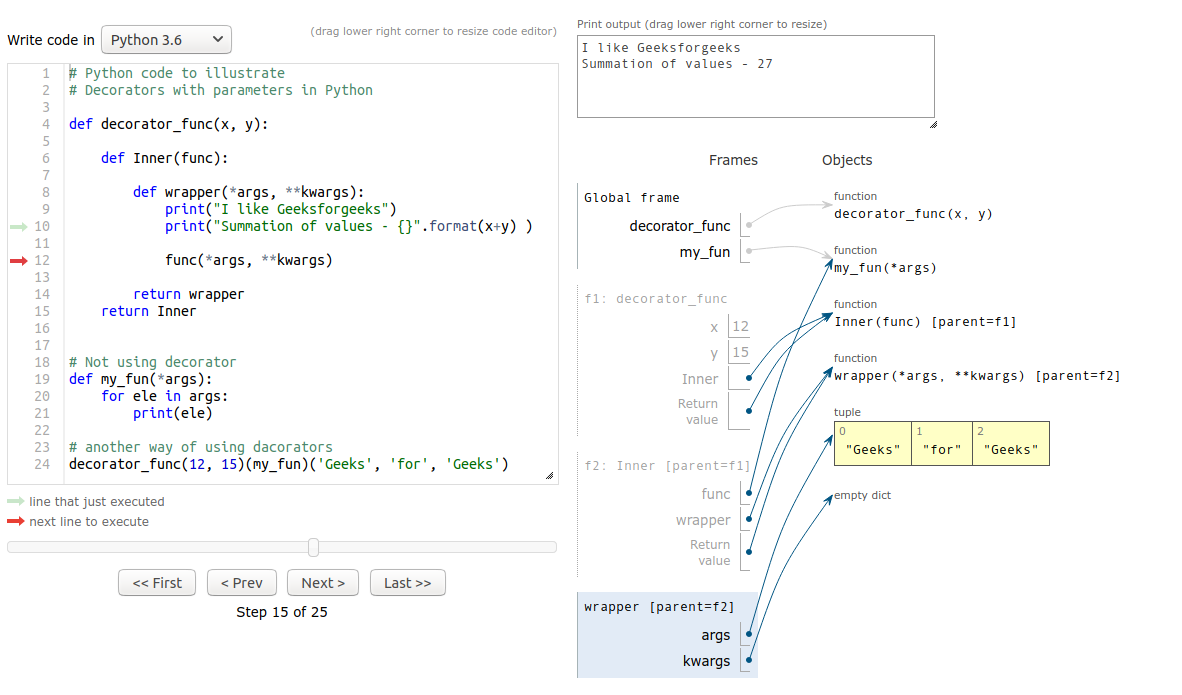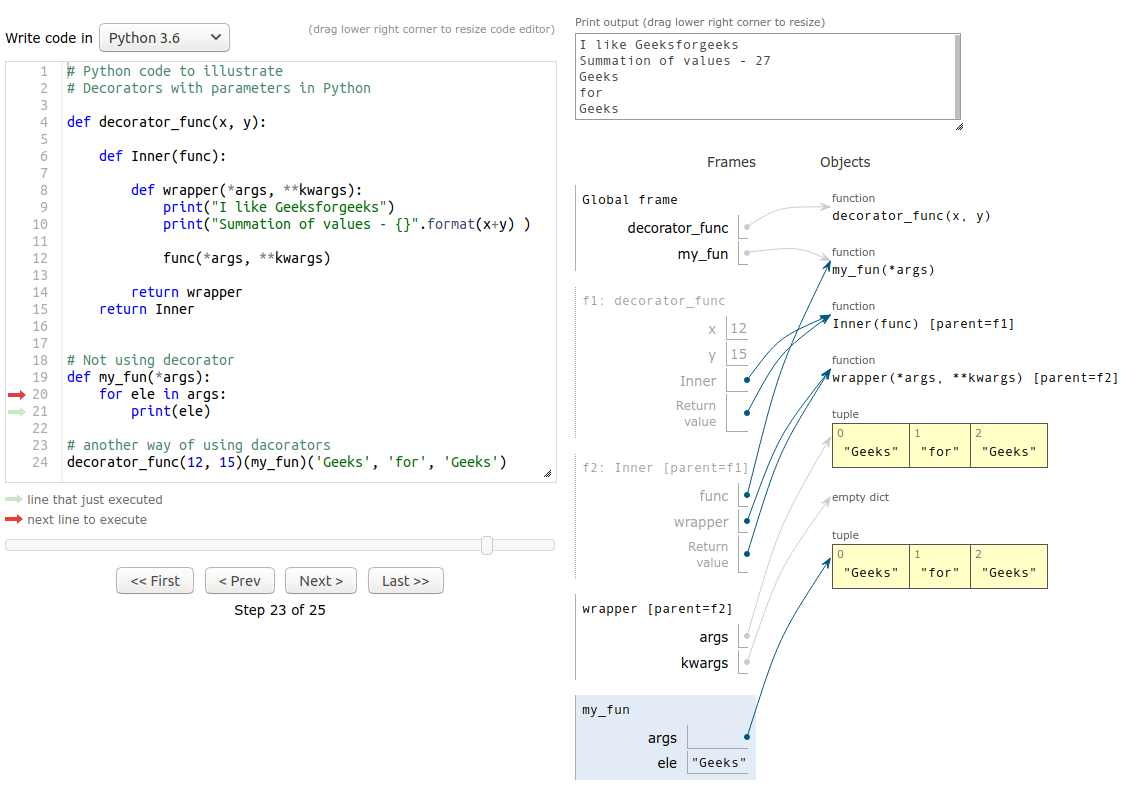# Decorators with parameters in Python

• Difficulty Level : Hard
• Last Updated : 17 Nov, 2021

Prerequisite: Decorators in Python, Function Decorators

We know Decorators are a very powerful and useful tool in Python since it allows programmers to modify the behavior of function or class. In this article, we will learn about the Decorators with Parameters with help of multiple examples.
Python functions are First Class citizens which means that functions can be treated similarly to objects.

Attention geek! Strengthen your foundations with the Python Programming Foundation Course and learn the basics.

To begin with, your interview preparations Enhance your Data Structures concepts with the Python DS Course. And to begin with your Machine Learning Journey, join the Machine Learning - Basic Level Course

• Function can be assigned to a variable i.e they can be referenced.
• Function can be passed as an argument to another function.
• Function can be returned from a function.

Decorators with parameters is similar to normal decorators.

## The syntax for decorators with parameters :

```@decorator(params)
def func_name():
''' Function implementation'''```

The above code is equivalent to

```def func_name():
''' Function implementation'''

func_name = (decorator(params))(func_name)
"""```

As the execution starts from left to right decorator(params) is called which returns a function object fun_obj. Using the fun_obj the call fun_obj(fun_name) is made. Inside the inner function, required operations are performed and the actual function reference is returned which will be assigned to func_name. Now, func_name() can be used to call the function with decorator applied on it.

How Decorator with parameters is implemented

## Python3

 `def` `decorators(``*``args, ``*``*``kwargs):` `    ``def` `inner(func):` `        ``'''` `           ``do operations with func` `        ``'''` `        ``return` `func` `    ``return` `inner ``#this is the fun_obj mentioned in the above content`   `@decorators``(params)` `def` `func():` `    ``"""` `         ``function implementation` `    ``"""`

Here params can also be empty.

Observe these first :

## Python3

 `# Python code to illustrate ` `# Decorators basic in Python `   `def` `decorator_fun(func): ` `  ``print``(``"Inside decorator"``) `   `  ``def` `inner(``*``args, ``*``*``kwargs): ` `    ``print``(``"Inside inner function"``) ` `    ``print``(``"Decorated the function"``) ` `    ``# do operations with func` `    `  `    ``func()` `    `  `  ``return` `inner `   `@decorator_fun` `def` `func_to(): ` `    ``print``(``"Inside actual function"``) `   `func_to() `

Another Way:

## Python3

 `# Python code to illustrate ` `# Decorators with parameters in Python `   `def` `decorator_fun(func): ` `  ``print``(``"Inside decorator"``) `   `  ``def` `inner(``*``args, ``*``*``kwargs): ` `    ``print``(``"Inside inner function"``) ` `    ``print``(``"Decorated the function"``) ` `    `  `    ``func()` `    `  `  ``return` `inner `     `def` `func_to(): ` `    ``print``(``"Inside actual function"``) `   `# another way of using decorators` `decorator_fun(func_to)() `

Output:

```Inside decorator
Inside inner function
Decorated the function
Inside actual function```

Let’s move to another example:

Example #1:

## Python3

 `# Python code to illustrate ` `# Decorators with parameters in Python `   `def` `decorator(``*``args, ``*``*``kwargs):` `    ``print``(``"Inside decorator"``)` `    `  `    ``def` `inner(func):` `        `  `        ``# code functionality here` `        ``print``(``"Inside inner function"``)` `        ``print``(``"I like"``, kwargs[``'like'``]) ` `        `  `        ``func()` `        `  `    ``# returning inner function    ` `    ``return` `inner`   `@decorator``(like ``=` `"geeksforgeeks"``)` `def` `my_func():` `    ``print``(``"Inside actual function"``)`

Output:

```Inside decorator
Inside inner function
I like geeksforgeeks
Inside actual function```

Example #2:

## Python3

 `# Python code to illustrate ` `# Decorators with parameters in Python `   `def` `decorator_func(x, y):`   `    ``def` `Inner(func):`   `        ``def` `wrapper(``*``args, ``*``*``kwargs):` `            ``print``(``"I like Geeksforgeeks"``)` `            ``print``(``"Summation of values - {}"``.``format``(x``+``y) )`   `            ``func(``*``args, ``*``*``kwargs)` `            `  `        ``return` `wrapper` `    ``return` `Inner`     `# Not using decorator ` `def` `my_fun(``*``args):` `    ``for` `ele ``in` `args:` `        ``print``(ele)`   `# another way of using decorators` `decorator_func(``12``, ``15``)(my_fun)(``'Geeks'``, ``'for'``, ``'Geeks'``)`

Output:

```I like Geeksforgeeks
Summation of values - 27
Geeks
for
Geeks```

This example also tells us that Outer function parameters can be accessed by the enclosed inner function.

Example #3:

## Python3

 `# Python code to illustrate` `# Decorators with parameters in Python  (Multi-level Decorators)`     `def` `decodecorator(dataType, message1, message2):` `    ``def` `decorator(fun):` `        ``print``(message1)` `        ``def` `wrapper(``*``args, ``*``*``kwargs):` `            ``print``(message2)` `            ``if` `all``([``type``(arg) ``=``=` `dataType ``for` `arg ``in` `args]):` `                ``return` `fun(``*``args, ``*``*``kwargs)` `            ``return` `"Invalid Input"` `        ``return` `wrapper` `    ``return` `decorator`     `@decodecorator``(``str``, ``"Decorator for 'stringJoin'"``, ``"stringJoin started ..."``)` `def` `stringJoin(``*``args):` `    ``st ``=` `''` `    ``for` `i ``in` `args:` `        ``st ``+``=` `i` `    ``return` `st`     `@decodecorator``(``int``, ``"Decorator for 'summation'\n"``, ``"summation started ..."``)` `def` `summation(``*``args):` `    ``summ ``=` `0` `    ``for` `arg ``in` `args:` `        ``summ ``+``=` `arg` `    ``return` `summ`     `print``(stringJoin(``"I "``, ``'like '``, ``"Geeks"``, ``'for'``, ``"geeks"``))` `print``()` `print``(summation(``19``, ``2``, ``8``, ``533``, ``67``, ``981``, ``119``))`

Output:

```Decorator for 'stringJoin'
Decorator for 'summation'

stringJoin started ...
I like Geeksforgeeks

summation started ...
1729```

1. Inside the Decorator2. Inside the functionNote: Image snapshots are taken using PythonTutor.

My Personal Notes arrow_drop_up
Recommended Articles
Page :